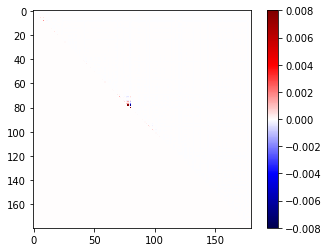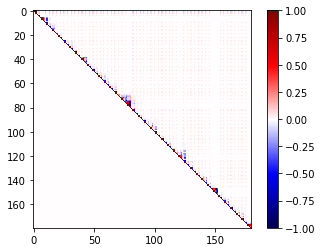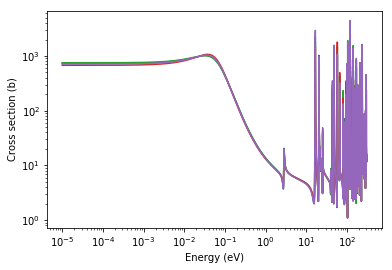# Nuclear Data: Resonance Covariance¶

nuclear-data-resonance-covariance

In this notebook we will explore features of the Python API that allow us to import and manipulate resonance covariance data. A full description of the ENDF-VI and ENDF-VII formats can be found in the ENDF102 manual.

In :
%matplotlib inline
import os
from pprint import pprint
import shutil
import subprocess
import urllib.request

import h5py
import numpy as np
import matplotlib.pyplot as plt

import openmc.data


### ENDF: Resonance Covariance Data¶

Let's download the ENDF/B-VII.1 evaluation for $^{157}$Gd and load it in:

In :
# Download ENDF file
url = 'https://t2.lanl.gov/nis/data/data/ENDFB-VII.1-neutron/Gd/157'

gd157_endf = openmc.data.IncidentNeutron.from_endf(filename, covariance=True)
gd157_endf

Out:
<IncidentNeutron: Gd157>

We can access the parameters contained within File 32 in a similar manner to the File 2 parameters from before.

In :
gd157_endf.resonance_covariance.ranges.parameters[:5]

Out:
energy J neutronWidth captureWidth fissionWidthA fissionWidthB L
0 0.0314 2.0 0.000474 0.1072 0.0 0.0 0
1 2.8250 2.0 0.000345 0.0970 0.0 0.0 0
2 16.2400 1.0 0.000400 0.0910 0.0 0.0 0
3 16.7700 2.0 0.012800 0.0805 0.0 0.0 0
4 20.5600 2.0 0.011360 0.0880 0.0 0.0 0

The newly created object will contain multiple resonance regions within gd157_endf.resonance_covariance.ranges. We can access the full covariance matrix from File 32 for a given range by:

In :
covariance = gd157_endf.resonance_covariance.ranges.covariance


This covariance matrix currently only stores the upper triangular portion as covariance matrices are symmetric. Plotting the covariance matrix:

In :
plt.imshow(covariance, cmap='seismic',vmin=-0.008, vmax=0.008)
plt.colorbar()

Out:
<matplotlib.colorbar.Colorbar at 0x14cf90c73550>The correlation matrix can be constructed using the covariance matrix and also give some insight into the relations among the parameters.

In :
corr = np.zeros([len(covariance),len(covariance)])
for i in range(len(covariance)):
for j in range(len(covariance)):
corr[i, j]=covariance[i, j]/covariance[i, i]**(0.5)/covariance[j, j]**(0.5)
plt.imshow(corr, cmap='seismic',vmin=-1.0, vmax=1.0)
plt.colorbar()

Out:
<matplotlib.colorbar.Colorbar at 0x14cf90b682e8>### Sampling and Reconstruction¶

The covariance module also has the ability to sample a new set of parameters using the covariance matrix. Currently the sampling uses numpy.multivariate_normal(). Because parameters are assumed to have a multivariate normal distribution this method doesn't not currently guarantee that sampled parameters will be positive.

In :
rm_resonance = gd157_endf.resonances.ranges
n_samples = 5
samples = gd157_endf.resonance_covariance.ranges.sample(n_samples)
type(samples)

/home/romano/openmc/openmc/data/resonance_covariance.py:233: UserWarning: Sampling routine does not guarantee positive values for parameters. This can lead to undefined behavior in the reconstruction routine.
warnings.warn(warn_str)

Out:
openmc.data.resonance.ReichMoore

The sampling routine requires the incorporation of the openmc.data.ResonanceRange for the same resonance range object. This allows each sample itself to be its own openmc.data.ResonanceRange with a new set of parameters. Looking at some of the sampled parameters below:

In :
print('Sample 1')
samples.parameters[:5]

Sample 1

Out:
energy L J neutronWidth captureWidth fissionWidthA fissionWidthB
0 0.030679 0 2.0 0.000473 0.108576 0.0 0.0
1 2.823843 0 2.0 0.000351 0.086418 0.0 0.0
2 16.281147 0 1.0 0.000458 0.106825 0.0 0.0
3 16.771760 0 2.0 0.013598 0.072837 0.0 0.0
4 20.561545 0 2.0 0.011164 0.086616 0.0 0.0
In :
print('Sample 2')
samples.parameters[:5]

Sample 2

Out:
energy L J neutronWidth captureWidth fissionWidthA fissionWidthB
0 0.032858 0 2.0 0.000479 0.105208 0.0 0.0
1 2.823859 0 2.0 0.000361 0.093748 0.0 0.0
2 16.203069 0 1.0 0.000264 0.015233 0.0 0.0
3 16.765055 0 2.0 0.013648 0.076119 0.0 0.0
4 20.557679 0 2.0 0.011140 0.097548 0.0 0.0

We can reconstruct the cross section from the sampled parameters using the reconstruct method of openmc.data.ResonanceRange. For more on reconstruction see the Nuclear Data example notebook.

In :
gd157_endf.resonances.ranges

Out:
[<openmc.data.resonance.ReichMoore at 0x14cf93855d68>,
<openmc.data.resonance.Unresolved at 0x14cf938c02e8>]
In :
energy_range = [rm_resonance.energy_min, rm_resonance.energy_max]
energies = np.logspace(np.log10(energy_range),
np.log10(energy_range), 10000)
for sample in samples:
xs = sample.reconstruct(energies)
elastic_xs = xs
plt.loglog(energies, elastic_xs)
plt.xlabel('Energy (eV)')
plt.ylabel('Cross section (b)')

Out:
Text(0, 0.5, 'Cross section (b)')### Subset Selection¶

Another capability of the covariance module is selecting a subset of the resonance parameters and the corresponding subset of the covariance matrix. We can do this by specifying the value we want to discriminate and the bounds within one energy region. Selecting only resonances with J=2:

In :
lower_bound = 2;  # inclusive
upper_bound = 2;  # inclusive
rm_res_cov_sub = gd157_endf.resonance_covariance.ranges.subset('J',[lower_bound,upper_bound])
rm_res_cov_sub.file2res.parameters[:5]

Out:
energy L J neutronWidth captureWidth fissionWidthA fissionWidthB
0 0.0314 0 2.0 0.000474 0.1072 0.0 0.0
1 2.8250 0 2.0 0.000345 0.0970 0.0 0.0
3 16.7700 0 2.0 0.012800 0.0805 0.0 0.0
4 20.5600 0 2.0 0.011360 0.0880 0.0 0.0
5 21.6500 0 2.0 0.000376 0.1140 0.0 0.0

The subset method will also store the corresponding subset of the covariance matrix

In :
rm_res_cov_sub.covariance
gd157_endf.resonance_covariance.ranges.covariance.shape

Out:
(180, 180)

Checking the size of the new covariance matrix to be sure it was sampled properly:

In :
old_n_parameters = gd157_endf.resonance_covariance.ranges.parameters.shape
old_shape = gd157_endf.resonance_covariance.ranges.covariance.shape
new_n_parameters = rm_res_cov_sub.file2res.parameters.shape
new_shape = rm_res_cov_sub.covariance.shape
print('Number of parameters\nOriginal: '+str(old_n_parameters)+'\nSubet: '+str(new_n_parameters)+'\nCovariance Size\nOriginal: '+str(old_shape)+'\nSubset: '+str(new_shape))

Number of parameters
Original: 60
Subet: 36
Covariance Size
Original: (180, 180)
Subset: (108, 108)


And finally, we can sample from the subset as well

In :
samples_sub = rm_res_cov_sub.sample(n_samples)
samples_sub.parameters[:5]

Out:
energy L J neutronWidth captureWidth fissionWidthA fissionWidthB
0 0.030488 0 2.0 0.000473 0.108946 0.0 0.0
1 2.825944 0 2.0 0.000328 0.098328 0.0 0.0
2 16.773886 0 2.0 0.012984 0.076779 0.0 0.0
3 20.565737 0 2.0 0.011628 0.088958 0.0 0.0
4 21.646469 0 2.0 0.000389 0.127833 0.0 0.0